Search by Topic

Resources tagged with Patterned numbers similar to The Numbers Give the Design:

Filter by: Content type:
Age range:
Challenge level:

There are 47 results

Broad Topics > Numbers and the Number System > Patterned numbers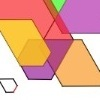The Numbers Give the Design

Age 7 to 11 Challenge Level:

Make new patterns from simple turning instructions. You can have a go using pencil and paper or with a floor robot.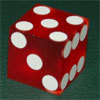Taking a Die for a Walk

Age 5 to 11 Challenge Level:

Investigate the numbers that come up on a die as you roll it in the direction of north, south, east and west, without going over the path it's already made.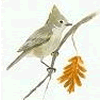Birds in the Garden

Age 5 to 11 Challenge Level:

This activity asks you to collect information about the birds you see in the garden. Are there patterns in the data or do the birds seem to visit randomly?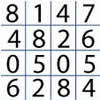Tables Without Tens

Age 7 to 11 Challenge Level:

Investigate and explain the patterns that you see from recording just the units digits of numbers in the times tables.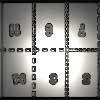Magazines

Age 7 to 11 Challenge Level:

Let's suppose that you are going to have a magazine which has 16 pages of A5 size. Can you find some different ways to make these pages? Investigate the pattern for each if you number the pages.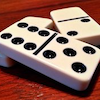Domino Sets

Age 7 to 11 Challenge Level:

How do you know if your set of dominoes is complete?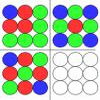Counter Ideas

Age 7 to 11 Challenge Level:

Here are some ideas to try in the classroom for using counters to investigate number patterns.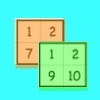Sorting the Numbers

Age 5 to 11 Challenge Level:

Complete these two jigsaws then put one on top of the other. What happens when you add the 'touching' numbers? What happens when you change the position of the jigsaws?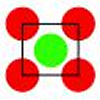Centred Squares

Age 7 to 11 Challenge Level:

This challenge, written for the Young Mathematicians' Award, invites you to explore 'centred squares'.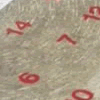All Seated

Age 7 to 11 Challenge Level:

Look carefully at the numbers. What do you notice? Can you make another square using the numbers 1 to 16, that displays the same properties?Sending Cards

Age 7 to 11 Challenge Level:

This challenge asks you to investigate the total number of cards that would be sent if four children send one to all three others. How many would be sent if there were five children? Six?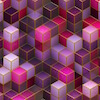Bundles of Cubes

Age 7 to 11 Challenge Level:

Watch this animation. What do you notice? What happens when you try more or fewer cubes in a bundle?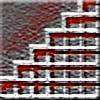Stairs

Age 5 to 11 Challenge Level:

This challenge is to design different step arrangements, which must go along a distance of 6 on the steps and must end up at 6 high.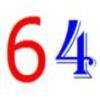It's All about 64

Age 7 to 11 Challenge Level:

Write the numbers up to 64 in an interesting way so that the shape they make at the end is interesting, different, more exciting ... than just a square.Month Mania

Age 5 to 11 Challenge Level:

Can you design a new shape for the twenty-eight squares and arrange the numbers in a logical way? What patterns do you notice?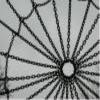Taking Steps

Age 7 to 11 Challenge Level:

In each of the pictures the invitation is for you to: Count what you see. Identify how you think the pattern would continue.Diagonal in a Spiral

Age 7 to 11 Challenge Level:

Investigate the totals you get when adding numbers on the diagonal of this pattern in threes.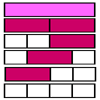Rods and Rods

Age 7 to 11 Challenge Level:

Using only the red and white rods, how many different ways are there to make up the other colours of rod?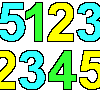Sept03 Sept03 Sept03

Age 7 to 11 Challenge Level:

This number has 903 digits. What is the sum of all 903 digits?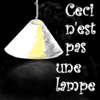Shedding Some Light

Age 7 to 11 Challenge Level:

Make an estimate of how many light fittings you can see. Was your estimate a good one? How can you decide?Colour Building

Age 11 to 14 Challenge Level:

Using only the red and white rods, how many different ways are there to make up the other colours of rod?Pyramids

Age 11 to 14 Challenge Level:

What are the missing numbers in the pyramids?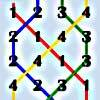Oranges and Lemons, Say the Bells of St Clement's

Age 11 to 14 Challenge Level:

Bellringers have a special way to write down the patterns they ring. Learn about these patterns and draw some of your own.Always the Same

Age 11 to 14 Challenge Level:

Arrange the numbers 1 to 16 into a 4 by 4 array. Choose a number. Cross out the numbers on the same row and column. Repeat this process. Add up you four numbers. Why do they always add up to 34?Top-heavy Pyramids

Age 11 to 14 Challenge Level:

Use the numbers in the box below to make the base of a top-heavy pyramid whose top number is 200.Swimming Pool Tiles

Age 7 to 11 Challenge Level:

This activity creates an opportunity to explore all kinds of number-related patterns.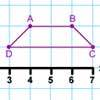Transformation Tease

Age 7 to 11 Challenge Level:

What are the coordinates of this shape after it has been transformed in the ways described? Compare these with the original coordinates. What do you notice about the numbers?Tower of Hanoi

Age 11 to 14 Challenge Level:

The Tower of Hanoi is an ancient mathematical challenge. Working on the building blocks may help you to explain the patterns you notice.Chameleons

Age 11 to 14 Challenge Level:

Whenever two chameleons of different colours meet they change colour to the third colour. Describe the shortest sequence of meetings in which all the chameleons change to green if you start with 12. . . .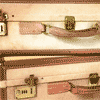Unlocking the Case

Age 7 to 11 Challenge Level:

A case is found with a combination lock. There is one clue about the number needed to open the case. Can you find the number and open the case?Score

Age 11 to 14 Challenge Level:

There are exactly 3 ways to add 4 odd numbers to get 10. Find all the ways of adding 8 odd numbers to get 20. To be sure of getting all the solutions you will need to be systematic. What about. . . .Like Powers

Age 11 to 14 Challenge Level:

Investigate $1^n + 19^n + 20^n + 51^n + 57^n + 80^n + 82^n$ and $2^n + 12^n + 31^n + 40^n + 69^n + 71^n + 85^n$ for different values of n.Small Change

Age 11 to 14 Challenge Level:

In how many ways can a pound (value 100 pence) be changed into some combination of 1, 2, 5, 10, 20 and 50 pence coins?Pattern Power

Age 5 to 14

Mathematics is the study of patterns. Studying pattern is an opportunity to observe, hypothesise, experiment, discover and create.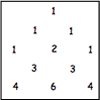Investigating Pascal's Triangle

Age 7 to 14 Challenge Level:

In this investigation, we look at Pascal's Triangle in a slightly different way - rotated and with the top line of ones taken off.Hidden Rectangles

Age 11 to 14 Challenge Level:

Rectangles are considered different if they vary in size or have different locations. How many different rectangles can be drawn on a chessboard?Sum Equals Product

Age 11 to 14 Challenge Level:

The sum of the numbers 4 and 1 [1/3] is the same as the product of 4 and 1 [1/3]; that is to say 4 + 1 [1/3] = 4 � 1 [1/3]. What other numbers have the sum equal to the product and can this be so for. . . .Age 11 to 14 Challenge Level:

A little bit of algebra explains this 'magic'. Ask a friend to pick 3 consecutive numbers and to tell you a multiple of 3. Then ask them to add the four numbers and multiply by 67, and to tell you. . . .Can You Find a Perfect Number?

Age 7 to 14

Can you find any perfect numbers? Read this article to find out more...How Many Miles to Go?

Age 11 to 14 Challenge Level:

How many more miles must the car travel before the numbers on the milometer and the trip meter contain the same digits in the same order?Squares, Squares and More Squares

Age 11 to 14 Challenge Level:

Can you dissect a square into: 4, 7, 10, 13... other squares? 6, 9, 12, 15... other squares? 8, 11, 14... other squares?Power Crazy

Age 11 to 14 Challenge Level:

What can you say about the values of n that make $7^n + 3^n$ a multiple of 10? Are there other pairs of integers between 1 and 10 which have similar properties?Four Coloured Lights

Age 11 to 14 Challenge Level:

Imagine a machine with four coloured lights which respond to different rules. Can you find the smallest possible number which will make all four colours light up?A One in Seven Chance

Age 11 to 14 Challenge Level:

What is the remainder when 2^{164}is divided by 7?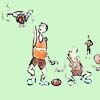One Basket or Group Photo

Age 7 to 18 Challenge Level:

Libby Jared helped to set up NRICH and this is one of her favourite problems. It's a problem suitable for a wide age range and best tackled practically.Lastly - Well

Age 11 to 14 Challenge Level:

What are the last two digits of 2^(2^2003)?Sept 03

Age 11 to 14 Challenge Level:

What is the last digit of the number 1 / 5^903 ?